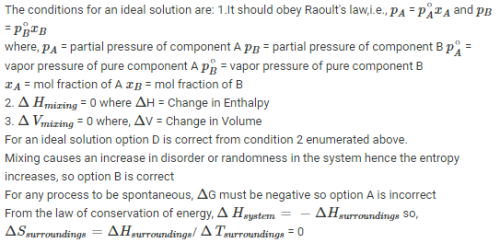Courses

# MCQ (Previous Year Questions) - Solutions (Level 2)

## 13 Questions MCQ Test Chemistry for JEE Advanced | MCQ (Previous Year Questions) - Solutions (Level 2)

Description
This mock test of MCQ (Previous Year Questions) - Solutions (Level 2) for JEE helps you for every JEE entrance exam. This contains 13 Multiple Choice Questions for JEE MCQ (Previous Year Questions) - Solutions (Level 2) (mcq) to study with solutions a complete question bank. The solved questions answers in this MCQ (Previous Year Questions) - Solutions (Level 2) quiz give you a good mix of easy questions and tough questions. JEE students definitely take this MCQ (Previous Year Questions) - Solutions (Level 2) exercise for a better result in the exam. You can find other MCQ (Previous Year Questions) - Solutions (Level 2) extra questions, long questions & short questions for JEE on EduRev as well by searching above.
QUESTION: 1

### The van't Hoff factor for 0.1 M Ba(NO3)2 solution is 2.74. The degree of dissociation is    [JEE 1999]

Solution: Ans hai b : i=1+ (n-1) @ 2.74=1+(3-1)@ 1.74/2=@ @=87%
QUESTION: 2

Solution:
QUESTION: 3

### During depression of freezing point in a solution, the following are in equilibrium     [JEE 2003]

Solution:
QUESTION: 4

A 0.004 M solution of Na2SO4 is isotonic with a 0.010 M solution of glucose at same temperature. The apparent degree of dissociation of Na2SO4 is : [JEE 2004]

Solution:
The reaction is given as,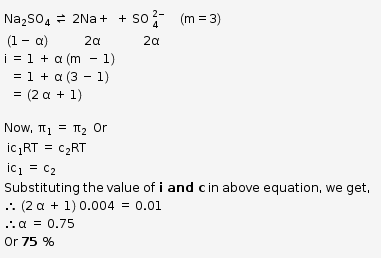QUESTION: 5

The elevation in boiling point, when 13.44 g of freshly prepared CuCl2 are added to one kilogram of water, is [Some useful data, Kb(H2O)= 0.52 kg K mol_1, mol. wt. of CuCl2= 134.4 gm ]      [JEE 2005]

Solution:
QUESTION: 6

When 20 g of naphtholic acid (C11H8O2) is dissolved in 50 g of benzene (Kf = 1.72 K kg mol_1), a freezing point depression of 2 K is observed. The van't Hoff factor (i) is :       [JEE 2007]

Solution:
QUESTION: 7

Properties such as boiling point, freezing point and vapour pressure of a pure solvent change when solute molecules are added to get homogeneous solution. These are called colligative properties. Applications of colligative properties are very useful in day-today life. One of its examples is the use of ethylene glycol and water mixture as anti-freezing liquid in the radiator of automobiles.

A solution M is prepared by mixing ethanol and water. The mole fraction of ethanol in the mixture is 0.9.

Given: Freezing point depression constant of water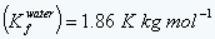Freezing point depression constant of ethanol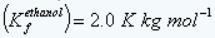Boiling point elevation constant of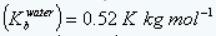Boiling point elevation constant of ethanol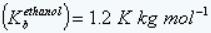Standard freezing point of water = 273 K
Standard freezing point of ethanol = 155.7 K
Standard boiling point of water = 373 K
Standard boiling point of ethanol = 351.5 K
Vapour pressure of pure water = 32.8 mm Hg
Vapour pressure of pure ethanol = 40 mm Hg
Molecular weight of water = 18 g mol−1
Molecular weight of ethanol = 46 g mol−1

In answering the following questions, consider the solutions to be ideal dilute solutions and solutes to be non-volatile and non-dissociative.     [JEE 2008]

The freezing point of the solution M is

Solution: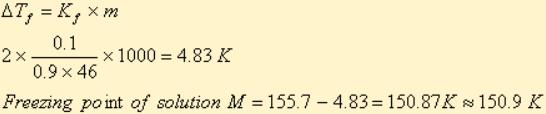QUESTION: 8

The vapour pressure of the solution M is

Solution:
QUESTION: 9

Water is added to the solution M such that the mole fraction of water in the solution becomes 0.9. The boiling point of this solution is:

Solution: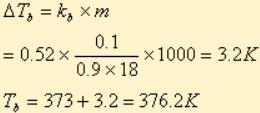QUESTION: 10

The Henry's law constant for the solubility of N2 gas in water at 298 K is 1.0 × 105atm. The mole fraction of N2 in air is 0.8. The number of moles of N2from air dissolved in 10 moles of water at 298 K and 5 atm pressure is :       [JEE 2009]

Solution:
QUESTION: 11

The freezing point (in ºC) of a solution containing 0.1 g of K3[Fe(CN)6](Mol. Wt. 329) in 100 g of water (Kf = 1.86 K kg mol_1) is      [JEE 2011]

Solution:
QUESTION: 12

. For a dilute solution containing 2.5 g of a non-voltile non-electrolyte solute in 100 g of water, the elevation in boiling point at 1 atm pressure is 2ºC. Assuming concentration of solute is much lower than the concentration of solvent, the vapour pressure (mm of Hg) of the solution is (take Kb = 0.76 K kg mol_1) [JEE 2012]

Solution:
*Multiple options can be correct
QUESTION: 13

Benzene and naphthalene form an ideal solution at room temeprature. For this process the true statement(s) is (are)          [JEE 2013]

Solution: